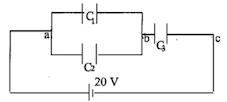Problem: Three capacitors C1 = 4µF, C2 = 8µF, and C3 = 6µF are connected across a 20V battery as shown.Find the voltage drop across C1.Find the voltage drop across C2.Find the voltage drop across C3.Find the charge on the capacitor C2.Find the charge on the capacitor C3.

83% (10 ratings)
Problem Details

Three capacitors C1 = 4µF, C2 = 8µF, and C3 = 6µF are connected across a 20V battery as shown.

1. Find the voltage drop across C1.
2. Find the voltage drop across C2.
3. Find the voltage drop across C3.
4. Find the charge on the capacitor C2.
5. Find the charge on the capacitor C3.